A GUIDE TO

POSITIVE MATRIX FACTORIZATION

Philip K. Hopke

Center for Air Resources Engineering and Science

Clarkson University

Potsdam, NY 13699-5708

INTRODUCTION

The fundamental principle of source/receptor relationships is that mass conservation can be assumed and a mass balance analysis can be used to identify and apportion sources of airborne particulate matter in the atmosphere. This methodology has generally been referred to within the air pollution research community as receptor modeling [Hopke, 1985; 1991]. The approach to obtaining a data set for receptor modeling is to determine a large number of chemical constituents such as elemental concentrations in a number of samples. Alternatively, automated electron microscopy can be used to characterize the composition and shape of particles in a series of particle samples. In either case, a mass balance equation can be written to account for all m chemical species in the n samples as contributions from p independent sources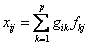(1)

where xij is the ith elemental concentration measured in the jth sample, fik is the gravimetric concentration (ng mg-1) of the ith element in material from the kth source, and gkj is the airborne mass concentration (mg m-3) of material from the kth source contributing to the jth sample.

There exist a set of natural physical constraints on the system that must be considered in developing any model for identifying and apportioning the sources of airborne particle mass [Henry, 1991]. The fundamental, natural physical constraints that must be obeyed are:

1)         The original data must be reproduced by the model; the model must explain the observations.

2)         The predicted source compositions must be non-negative; a source cannot have a negative percentage of an element.

3)         The predicted source contributions to the aerosol must all be non-negative; a source cannot emit negative mass.

4)         The sum of the predicted elemental mass contributions for each source must be less than or equal to total measured mass for each element; the whole is greater than or equal to the sum of its parts.

When developing and applying these models, it is necessary to keep these constraints in mind in order to be certain of obtaining physically realistic solutions.

The critical question is then what information is available to solve equation (1). It is assumed that the ambient concentrations of a series of chemical species have been measured for a set of particulate matter samples so that the xij values are always known. If the sources that contribute to those samples can be identified and their compositional patterns measured, then only the contributions of the sources to each sample need to be determined. These calculations are generally made using the effective variance least squares approach incorporated into the EPA’s CMB model. However, for many locations, the sources are either unknown or the compositions of the local particulate emissions have not been measured. Thus, it is desirable to estimate the number and compositions of the sources as well as their contributions to the measured PM. The multivariate data analysis methods that are used to solve this problem are generally referred to as factor analysis.

FACTOR ANALYSIS

The factor analysis problem can be visualized with the following example. Suppose a series of samples are taken in the vicinity of a highway where motor vehicles are using leaded gasoline and a steel mill making specialty steels. For these samples, measurements of Pb, Br, and Cr are made. This set of data can then be plotted in a three dimensional space as in Figure 1. A cloud of points can be observed.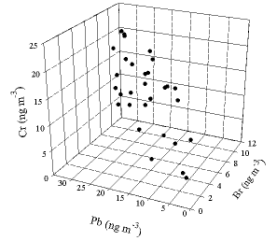Figure 1. Three dimensional plot of simulated data.

However, it is known that there are only two particle sources. The problem is then to determine the true dimensionality of the data and the relationships among the measured variables. That is goal of a factor analysis. In the case of this example, the relationships can be observed with a simple rotation of the axes so that we look down onto the figure so that the Cr axis sticks out of the page. This view is seen in Figure 2. Now it can be seen that the data really cluster around a line that represents the Pb-Br relationship in the particles emitted by the motor vehicles. The Cr values are distributed vertically and are independent of the other two elements. Factor analysis of this problem would find two sources and provide the relationship between the lead and bromine.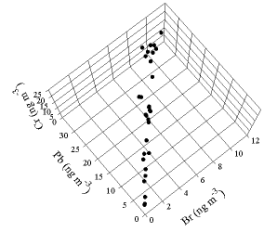Figure 2. Plot of the simulated data as viewed from above relative to the view in Figure 1.

.

Principal Component Analysis

The most common form of factor analysis is Principal Components Analysis (PCA). This method is generally available in most computer packages for statistical analysis. The PCA results are generally calculated using an eigenvector analysis of a correlation matrix [Hopke, 1985; Henry, 1991]. However, there are several problems associated with such methods [Henry, 1987; Paatero and Tapper, 1993, 1994]. The PCA model is based on a series of n samples in which m chemical species have been measured. Thus, equation (1) can be written in matrix form as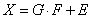(2)

The X matrix can also be defined in terms of the singular value decomposition.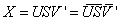(3)

where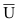and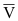are the first p columns of the U and V matrices. The U and V matrices are calculated from eigenvalue-eigenvector analyses of the XX’ and X’X matrices, respectively. It can be shown [Lawson and Hanson, 1974; Malinowski, 1991] that the second term on the right side of equation (3) estimates X in the least-squares sense that it gives the lowest possible value for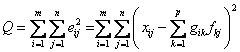(4)

The problem can be solved, but it does not produce a unique solution. It is possible to include a transformation into the equation.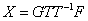where T is one of the potential infinity of transformation matrices. This transformation is called a rotation and is generally included in order to produce factors that appear to be closer to physically real source profiles.

To illustrate this problem, Figure 3 shows simulated data for ambient samples that consist of mixtures of the soil and basalt source profiles [Currie et al., 1984].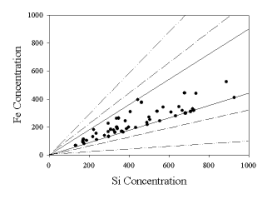Figure 3. Simulated data showing multiple possible source “profiles”

that could be used to fit the data.

This figure shows a plot of the iron and silicon values for a series of simulated samples. There need to be two profiles to reproduce each data point, but these “profiles” could range from the original axes to any of the other pairs of lines. Because there are no zero valued source contributions, the solid lines are not the “true” profiles. The true source profiles lie somewhere between the inner solid lines that enclose all of the points and the original axes, but without additional information, these profiles cannot be fully determined. This is the problem that was presented by Henry  when he described factor analysis as “ill-posed.”

Paatero and Tapper  have shown that in effect in PCA, there is scaling of the data by column or by row and that this scaling will lead to distortions in the analysis. They further show that the optimum scaling of the data would be to scale each data point individually so as to have more precise data having more influence on the solution than points that have higher uncertainties. However, they show that point-by-point scaling results in a scaled data matrix that cannot be reproduced by a conventional factor analysis based on the singular value decomposition. Thus, an alternative formulation of the factor analysis problem is needed.

Positive Matrix Factorization

Mathematical Framework

This new approach to factor analysis is Positive Matrix Factorization (PMF). In this new method, the problem of non-optimal scaling has been explicitly addressed. In order to properly scale the data, it is necessary to look explicitly at the problem as a least-squares problem. To begin this analysis, the elements of the "Residual Matrix," E are defined as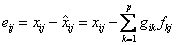(6)

where   i =1,..., m elements

j =1,..., n samples

k=1,..., p sources

An "object function," Q, that is to be minimized as a function of G and F is given by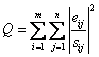(7)

where sij is an estimate of the “uncertainty” in the ith variable measured in the jth sample. One of the critical aspects of using the PMF method is the choice of these uncertainties and this process will be discussed in more detail below. The factor analysis problem is then to minimize Q(E) with respect to G and F with the constraint that each of the elements of G and F is to be non-negative.

Initially the problem was solved iteratively using alternating least squares  [Paatero and Tapper, 1993]. In alternating least-squares, one of the matrices, G or F, is taken as known and the chi-squared is minimized with respect to the other matrix as a weighted linear-least-squares problem. Then the roles of G and F are reversed so that the matrix that has just been calculated is fixed and the other is calculated by minimizing Q. The process then continues until convergence. However, this process can be slow.

In order to improve performance, each step in the iteration was modified so that modifications are made to both matrices [Paatero and Tapper, 1994]. Subsequently, Paatero  describes a global optimization scheme in which the joint solution is directly determined. This program, PMF2, is able to simultaneous vary the elements of G and F in each iterative step. The model will be designated as PMF while the program used to solve the model will be designated PMF2.

In PMF2, the non-negativity constraint is imposed through the use of a penalty function in an enhanced function, . Regularization can also be imposed to reduce rotational freedom so that the enhanced object function is defined asWhere P(G) and P(F) are penalty functions that prevent the factors G and F from becoming negative. R(G) and R(F) are regularization functions that remove some of the rotational indeterminacy. For the unconstrained case, α and ß are set to zero. However, the regularization terms are always present in the analysis. All of the coefficients, α, β, γ, and δ, are given smaller values during the iterations so that their final values are negligible but not zero. In practice, the log functions are approximated by a Taylor series expansion up to quadratic terms. The algorithm can obtain transient negative values, but it immediately adjusts the Taylor expansion so that the user never sees the negative values. Only non-negative values are stored in the factor matrices.

Subsequently, it was found useful to have a more versatile program for solving a more general set of problems. Xie et al. [1999b] found that a model that includes both terms like equation (1) and trilinear terms (product of 3 factors) provided a better fit to the data. Paatero  has developed a second program, the Multilinear Engine (ME), that can also solve the positive matrix factorization problem but with an approach that provides more flexibility in specifying the model. There are differences in the computational approach between PMF2 and ME. At this time, PMF2 has been more widely used and tested, but recent work with ME has shown the value of being able to build more complex data analysis models.

Estimation of Weights

The solution to the PMF problem using either program depends on estimating uncertainties for each of the data values used in the PMF analysis. There are three types of values that are typically available. Most of the data points have values that have been determined, xij, and their associated uncertainties, σij. One of the values of nuclear analytical methods is that it is easy to provide an estimate of the uncertainty associated with the measurement of each element in each sample. These uncertainties then serve as a starting point for the weight estimation.

There are samples in which the particular species cannot be observed because the concentration is below the method detection limit. Finally there are samples for which the values were not determined. These latter two types of data are often termed “missing” data. However, there are qualitative differences between them. In the below detection limit samples, the value is known to be small, but the exact concentration is not known. In the case where values could not be determined, the value is totally unknown.

Polissar et al.  has suggested the following for the concentration values and their associated error estimates: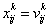For determined values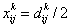For below limit of detection values                                              (9)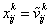For missing values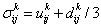For determined values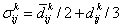For below limits of detection values                                            (10)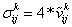For missing values

Where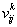,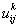, and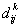are the measured concentration, the analytical uncertainty, and the method detection limit, respectively, for sample i, element j, and sampling site k,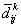is arithmetic mean of the detection limit value for the element j and the sampling site k; and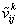is the geometric mean of the measured concentration of the element j at sampling site k.  Half of the average detection limits were used for below detection limits values in the calculations of the geometric means.

The detection limits specified the error estimates for low concentration while uncertainties provided the estimation of errors for high concentration of element j. Error estimates for the determined values were usually less than 50%; were used for below detection limits values and corresponding relative error estimates from 100% to 250% were used for these values according to equation (10). Geometric mean values with the corresponding error estimates equal to 4 times these values were used for the missing values so that relative error estimates for missing values were equal to 400%. Thus, both the values that were used for the below detection limit values and the values that were used for missing values had much lower weights in comparison to actually measured values.

“Missing” means that some elemental concentrations are missing for a specific sample but the concentration of at least one element had been reported for the particular sample. Then the missing concentrations and corresponding error estimates of other elements were calculated according to equations (9) and (10). Alternatively, heuristic error estimates, sij, for a given xij can be estimated based on the data point and its original error estimate, σij. The general equation is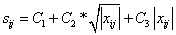(11)

with

C1 =   σij

C2 =   0 except for Poissonian processes

C3 =    dimensionless values between 0.1 – 0.2 are typically chosen so that the relative uncertainty of each data set was reasonable.

Similar approaches were used for estimating weights in other recent applications [Yakovleva et al., 1999; Chueinta et al., 2000].

PMF also offers an option when it is not possible to fully specify the individual data point errors. Models can be developed for this problem using a distribution that is known a priori. For environmental data, a lognormal model is available in which the user has to specify the logarithm of the "geometrical standard deviation" (log(GSD)) for each measured value. It is often sufficient to use the same value for all measured points if all of the values are truly lognormally distributed. However, zero values cannot occur in a lognormal distribution and yet such values can occur. These values make the factorization unreliable if they are processed under the assumption of pure lognormality. Then the assumption of lognormality needs to be modified. PMF offers the option to assume that there is an additional normal error superimposed onto the lognormal distribution. The method detection limit can be used as this additional error. The statistical properties of this model are not fully known. The expectation value of Q does not equal the degrees of freedom in lognormal model. However, PMF provides an estimate of the expected value of Q

Another important aspect of weighting of data points is the handling of extreme values. Environmental data typically shows a positively skewed distribution and often with a heavy tail. Thus, there can be extreme values in the distribution as well as true “outliers.” In either case, such high values would have significant influence on the solution (commonly referred to as leverage). This influence will generally distort the solution and thus, an approach to reduce their influence can be a useful tool. Thus, PMF offers a “robust” mode. The robust factorization based on the Huber influence function [Huber, 1981] is a technique of iterative reweighing of the individual data values. The least squares formulation, thus, becomes to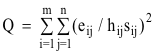(12)

where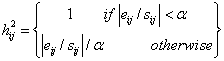α = the outlier distance and the value of α = 4.0 was chosen

It is generally advisable to use the robust mode when analyzing environmental data.

Our experience has generally found that the lognormal model and the robust mode provide the best results for typical particulate composition data. For the NOAA aerosol data from Barrow [Polissar et al., 1999], it was found that the lognormal model produced reasonable results but that the robust mode caused problems in terms of results that made physical sense. However, these data consist of monthly mean particle counts and light scattering efficiency and apparently behave somewhat differently from typical particle composition data.

Algorithms

Over the past several years several approaches to solving the PMF problem have been developed. Initially, a program called PMF2 utilized a unique algorithms [Paatero, 1997] for solving the factor analytic task. For small and medium-sized problems, this algorithm was found to be more efficient than ALS methods [Hopke et al., 1998]. It has now been applied to a large number of problems [Anderson et al., 2001;2002; Buzcu et al., 2003; Begum et al., 2004; Chueinta et al., 2000; Claiborn et al., 2002; Gao et al.2003; Hien et al., 2004; Huang et al., 1999; Kim and Hopke, 2004a&b; Kim et al., 2003a&b; 2004a&b; Larsen and Baker, 2003; Lee et al., 1999; 2002; 2003; Maykut et al., 2003; Miller et al., 2002; Paterson et al., 1999; Polissar et al., 1996; 1998; 1999; 2001; Prendes et al., 1999; Qin and Oduyemi, 2003; Qin et al., 2002; Ramadan et al., 2000; Xie et al., 1999b]. Huang et al.  analyzed elemental composition data for particulate matter samples collected at Narragansett, R.I. using both PMF and conventional factor analysis. They were able to resolve more components with more physically realistic compositions with PMF. Thus, the PMF approach has some inherent advantages particularly through its ability to individually weight each data point. PMF is somewhat more complex and harder to use, but it provides improved resolution of sources and better quantification of impacts of those sources than PCA [Huang et al., 1999].

Subsequently, an alternative approach that provides a flexible modeling system has been developed for solving the various PMF factor analytic least squares problems [Paatero, 1999]. This approach, called the multilinear engine (ME), has been applied to an environmental problem [Xie et al., 1999a] and has only recently been applied to a limited number of complex modeling problems [Chueinta et al., 2004; Hopke et al., 2003; Kim et al., 2003c; Paatero and Hopke, 2002; Paatero et al., 2003].

Guidance on the Use of PMF2

Initial Data Evaluation

There are severe limits to the extent that standard routines can be developed to pre-process complex airborne particulate matter compositional data sets. In our extensive experience in exploring a large number of data sets, there are unique aspects to virtually every data set. Thus, there will need to be clear caveats provided to the user of these procedures to indicate that data evaluation is not something that can be fully accomplished with standard procedures.

Variables can be categorized into several categories [Paatero and Hopke, 2003]. A variable will be called “a weak variable” if it contains signal and noise in comparable amounts. Similarly, variables containing much more noise than signal are termed “bad variables”. It is problematic to give precise definitions of the terms weak and bad variables. On one hand, currently there is not much practical experience about the suggested techniques. On the other hand, the shapes of distributions will be different for different variables. This should be taken into account when defining what weak or bad means. Also, the definition will depend on the representation of smallest concentrations: are they given as measured, or are they censored so that only the detection level (DL) is given instead of such actual values that are “Below Detection Level” (BDL).

The signal to noise ratio is calculated as defined in Paatero and Hopke (2003).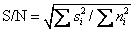(13)

A variable is defined to be weak if its S/N is between 0.2 and 2. If S/N < 0.2, then a variable is defined to be bad. As already suggested, these limits are somewhat arbitrary. The intention is that in a weak variable, there are similar amounts of signal and noise, while there is clearly less signal than noise in a bad variable.

For censored data, a tentative definition is based on the number mDLj of BDL values in column j. Denote the (average) detection limit for censored values in column j by δj. Then variable j is defined to be weak if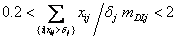(14)

An example of this definition: assume that 50% of values xij are BDL. Denote by Xj the average of those values xij that are above δj. Then Equation (14) reduces to 0.2< Xjj <2. Based on the initial work of Paatero and Hopke (2003), the following recommendation is made.

In PMF, an uncertainty is specified for each individual data value. If these uncertainties are specified too small or too large in comparison to the true error level of a certain variable, then an overweighting or downweighting of the variable occurs. Regarding weak/bad variables, the main result of this work is that even a small amount of overweighting is quite harmful and should be avoided. In contrast, moderate downweighting, by a factor of 2 or 3, never hurts much and sometimes is useful. Thus, it is recommended to routinely downweight all weak variables by a factor of 2 or 3. This practice will act as insurance and protect against occasions when the error level of some variables has been underestimated resulting in a risk of overweighting such variables. Regarding bad variables (where hardly any signal is visible from the noise), the recommendation is that such variables be entirely omitted from the model. If this is not desirable, then such variables should be strongly downweighted, by a factor of 5 or 10.

The procedure just described provides a overall view of a variable. It is also necessary to examine individual data values. Typically one examines the time series of the individual input variables looking for unusual patterns or values in the data. Similarly, the complete set of variable biplots should be plotted and explored. These can been examined to identify unusual points and potential relationships that should appear in the extracted factors.

Estimation of the Number of Factors

One of the most difficult issues in factor analysis is the determination of the number of factors to use in the model. There is no unequivocal method to identify the correct number of factors. There are a number of guidelines that can be used included the extracted Q-value, the nature of the scaled residual distributions, and the interpretability of the extracted factors.

The theoretical Q-value should be approximately equal to the number of degree of freedom, or approximately equal to the total number of data points in the data array. If the errors are properly estimated, then it can be seen that fitting each data point such that the reproduced value to within the estimated error value will contribute a value of approximately 1 to the Q value. Thus, it is tempting to examine the estimated Q value as a function of the number of factors to determine the number of factors to retain. However, this approach can be misleading if the data point uncertainties are not well determined. This problem has been observed when only the measured uncertainties are used as the weights. This problem can be minimized by using weights like are presented in Equation 10 or using a model for the data point errors as described in Equation 11. It is useful to look at the changes in Q as additional factors are calculated since after an appropriate number of factors are included in the fit, additional factors will not result in significant further improvements in the Q-value. Such behavior can be observed in Yakovleva et al. . Thus, PMF suffers from the same difficulties in determining the correct number of factors as all other forms of factor analysis.

As one of the methods to determine the quality of the fit, the residuals, eij, are examined. Typically the distributions of the residuals are plotted for each measured species. It is desirable to have symmetric distributions and to have all of the residuals within + 2 standard deviations. If there is skewness or a larger spread in the residuals, then the number of factors should be reexamined.

One of the disadvantages of a least-squares approach is that it can yield multiple solutions depending on the initial starting point. As part of the PMF program, it is possible to initiate random values in the F and G matrices as the starting point for an analysis. It is generally advisable to perform the analysis several times (typically 5) to be certain that the same solution is obtained. It has been our experience that one of the tests of the best selection of the number of factors is that one does not obtain multiple solutions or obtains at most one alternative solution. With greater or fewer factors than optimum, multiple solutions are more often obtained.

Finally, the quality of the solution must be examined in terms of how easily can the factors be identified as source types and do the corresponding source contribution patterns make sense. Source profiles can be compared to those that exist in the literature or those that have been locally measured. In each data set there are often specific events that can be identified. For example, in areas where the weather can be divided into wet and dry seasons, there should be appropriate differences in the source contributions during these two periods. If there is known activities such as construction, wildfires, or major transboundary episodes, these events should appear in the time series record of contributions.

Factor Rotations

In general, this type of bilinear factor analysis will have rotational ambiguity. There will not be a unique solution even though there will be a global minimum in the least-squares fitting process. The addition of constraints can reduce the rotational freedom in the system, but non-negativity alone does not generally result in a unique solution. One of the key features of PMF is that the rotations are part of the fitting process and not applied after the extraction of the factors as is done in eigenvector-based methods. Rotational freedom can be reduced by having known zero values in either the G or F matrices and forcing the solution to those values. Since rotations really represent addition and subtractions [Paatero and Tapper, 1994], the combination of zero values and non-negativity constraints reduces the rotational ambiguity.

One of the ways that rotations can be controlled in PMF2 is by using the parameter FPEAK. By setting a non-zero value of FPEAK, the routine is forced to add one G vector to another and subtract the corresponding F factors from each other and thereby yield more physically realistic solutions. The method requires a complicated mathematical process which is not described here [see Paatero, 1997]. The degree of rotational freedom is reduced if there are real zeroed values in the true F and G matrices. Then for positive FPEAK, zeroes in the F matrix restrict subtractions since no resulting value can become negative. Alternatively, a negative FPEAK causes subtractions in the G matrix and those subtractions are restricted if there zeroes in the matrix. If there are a sufficient number of zero values both in F and in G, then the solution is rotationally unique: there are no possible rotations. However, this case is rare.

Experience for real data sets has shown that positive, non-zero FPEAK values generally yield more realistic results. There is no theoretical basis for choosing a particular value of FPEAK. However, it is important to examine the Q value. Since the rotation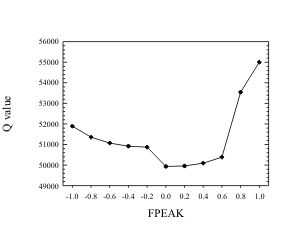Figure 4. Plot of Q as a function of FPEAK for a fine particle data set from Phoenix, AZ.

is integrated into the optimization scheme, the Q can change with the rotation. If the rotation forces the fit too away from the original Q, then the value of FPEAK should be moved toward zero. Figure 5 shows the behavior that has been observed for a number of data sets when Q is examined as a function of FPEAK. Typically the highest FPEAK value before the substantial rise in Q yielded the most physically interpretable source profiles. It has also been found in several cases that when examining the regression of the mass against the G values, FPEAK values can be varied to yield non-negative scaling factors while retaining physically reasonable source profiles.

The imposition of external information on the solution is another way in which PMF can control rotations. If specific values of profiles or time series are known to be zero, then it is possible to force the solution toward zero for those values through appropriate settings of Fkey and Gkey values. Details of setting up the input files are in the PMF User Guide.

When analyzing Hong Kong aerosol, Lee et al. found sulphate in almost all factors (Lee et al., 1999). Such compositions of factors did not appear plausible. The concentration of sulphate was then pulled down in a number of factors in another PMF2 run. The increase of Q was not significant and more plausible factors resulted from the pulled-down computations.

The analysis of Hong Kong data offers a simple example of pulling-down: only one compound is subjected to pulling. It would be possible to use pulling on several compounds, provided that there is reliable information about compounds that are not emitted by certain sources. In addition, it might also be possible to apply pulling-down to time series factors. Sometimes information about weather patters might reveal that a certain source cannot be present during certain time intervals. Then the time factor elements corresponding to such intervals could be pulled towards zero. Further discussion of the problem of rotation is provided by Paatero et al. . Research is in progress to provide additional guidance to address the rotational problem, but there are not yet definitive guidelines that can be applied to all data sets.

Error Estimates for the PMF Results

It is useful to have an estimate of the uncertainties in the elements of the F and G that have been calculated using PMF2. It is possible to estimate uncertainties using the process originally described by Roscoe and Hopke  and described in detail by Malinowski . The errors in the elements of one matrix are estimated based on the errors in the ambient concentration values and assuming that the other matrix is error-free. Each matrix (F or G) is treated similarly in turn such that an uncertainty is associated with each matrix element. Error models are currently under development that may produce better estimates for the PMF analysis results.

Mass Apportionment

The results of the PMF analysis reproduce the data and ensure that the source profiles and mass contributions are non-negative. However, it has not yet taken into account the measured mass. In addition the results are uncertain relative to a multiplicative scaling factor. Again a “1" can be introduced into equation 1.

If we have a sufficiently detailed chemical analysis such that those species that were not measured can either be assumed to be strongly correlated to measured species or represent sources that add negligible mass to the particulate matter samples, then the sum of the source contributions, gkj values, should be equal to the measured PM mass. Using this external information, the scaling constants, sk, can be determined by regressing the measured mass against the estimated source contribution values.

This regression provides several useful indicators of the quality of the solution. Clearly each of the sk values must be non-negative. If the regression produces a negative value, then it suggests that too many factors have been used. The source profiles need to be scaled by dividing the fik value by sk. Once the profiles are scaled, they can be summed and it can be determined if the sum of a source profile exceeds 100% (1000 ng/μg). In this case, it suggests that too few factors may have been chosen. Although it is desirable to require that the sk values be statistically significant, there can be weak or aperiodic sources for which these scaling coefficients may have high uncertainties.

Use of Complex Factor Analysis Models

To present the expanded factor analysis approach, the model is described from the viewpoint of one source, denoted by p. In reality, there are several sources and the observed concentrations are sums of contributions due to all sources, p=1,..., P. In the customary bilinear analysis, the contribution rijp of source p on day i to concentration of chemical species j is represented by the product gipfjp, where gip corresponds to the strength of source p on day i, and fjp corresponds to the concentration of compound j in the emission signature of source p.

In the present expanded PMF analysis, the bilinear equation (1) is augmented by another more complicated set of equations that contain modeling information. In its most basic form, the contribution rijp of source p is represented by the following expression: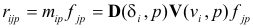The known values δi and vi indicate wind direction and wind speed on day i. The symbols D and V represent matrices, consisting of unknown values to be estimated during the fitting process. Their columns numbered p correspond to source number p. Because of typographic reasons, their indices are shown in parentheses, not as subscripts. The index value δi for day i is typically obtained by dividing the average wind direction of day i (in degrees) by ten and rounding to nearest integer. As an example, if source 2 comes strongly from the wind direction at 90°, then the element D(9,2) is likely to become large. The values vi are obtained from a chosen classification of wind speeds. The following classification was used in this work: 0 - 1.5 - 2.5 - 3.5 - 5.8 - ¥ m/s. Thus, vi=2 for such days when the average wind speed is between 1.5 and 2.5 m/s.

In component form, the equations of the model are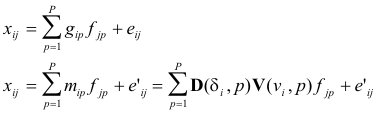(15)

The notation mip does not indicate a factor element to be determined, such as gip, but the expression defined by the physical model in question. In different physical models, mip will correspond to different expressions. Because the variability of mip is restricted by the model, the second set of equations (3) will produce a significantly poorer fit to the data than the first set of equations (3). The physical model, mip, is one of multiple possible models depending on the understanding of the system under study while the mass balance in the first set of equations should be much more applicable. Thus, the error estimates connected with the second set of equations must be (much) larger than the error estimates connected with the first set of equations.

The task of solving this expanded PMF model means that values of the unknown factor matrices G, F, D, and V are to be determined so that the model fits the data as well as possible. In other words, the sum-of-squares value Q, defined by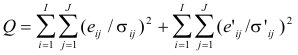(16)

is minimized with respect to the matrices G, F, D, and V, while the residuals eij and e’ij are determined by equations (16). The error estimates σ’ij must be specified (much) larger than the corresponding error estimates σij.

Since there are other sources of variation such as weekend/weekday source activity patterns or seasonal differences in emission rates or in atmospheric chemistry, additional factors are included in the model. In this case, wind direction, wind speed, time of year, and weekend/weekday will be used. In this case, 24 one-hour average values are available for wind speed and direction.  Time of year will be aggregated into six two-month periods or seasons, indicated for each day i by the index variables σi. (The Greek letter σ is used for two purposes: σij indicates the error estimates of data values, while σi indicates the season number for day i.). For the values i=1 to i=60, σi=1, meaning that January and February belong to the first season. For the values i=61 to i=121, σi=2, and so on.

Instead of the basic equation (15), the non-linear dependencies are now defined by the following multilinear expression: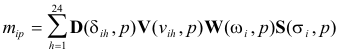(17)

where D(δih, p) is the element of D with the index for the wind direction during hour h of day i for the pth source, V(νih, p) is the element of V with the index for the wind speed during hour h of day i for the pth source, W(ωi, p) is the element of W with the index corresponding to day i for the weekday/weekend factor for the pth source, and S(σi, p) is the element of S with the index corresponding to the time-of-year classification of day i for the pth source. Each of these matrices, D, V, W, and S, contain unknown values to be estimated in the analysis. The specific factor elements used to fit a particular data point are selected based on the hourly (D, V) or daily (W, S) values of the corresponding variables. Thus, these auxiliary variables are not fitted, but serve as indicators to the values to be fitted.

The expanded model to be fitted consists thus of the basic bilinear equations plus a set of multilinear equations specifying the physical model: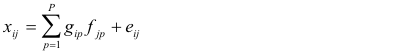(18)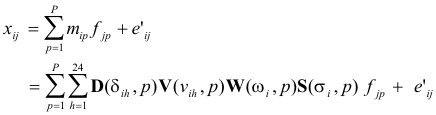(19)

The multilinear engine (ME) was used to solve this problem with non-negativity required for all of the elements of the matrices being estimated [Paatero, 1999].

This approach was first applied to simulated data previously used in an EPA workshop on advanced factor models and showed that the expanded model permitted more sources to be identified and accurately apportioned [Paatero and Hopke, 2002]. The wind direction factor was quite effective in pointing at the specific point sources contributing to the particulate matter mass.

It has now been applied to real data sets [Kim et al., 2003c]. In this study when compared to the standard PMF2 analysis of the same data [Kim et al., 2003a], the expanded model provides the extraction of additional factors. Particularly it appears to permit the separation of the contributions of spark-ignition (gasoline) from diesel vehicle emissions. This model has also been applied to high time resolution gaseous organic compound (VOC) data collected in Houston, TX [Zhao et al., 2004]. The wind direction factor appears to have been effective in pointing in the direction of known major sources of the observed VOCs. A number of other applications of this model are currently under development and are likely to be published in the near future.

In addition to this expanded version of the factor analysis model, the multilinear engine has been used to explore other models for data. Hopke et al.  have analyze a complex set of personal exposure data. The particle samples were collected with personal samplers (PEM) from 10 elderly subjects, their apartments, a central indoor location, and outdoors. The PEM data were analyzed using a complex model with a target for soil that included factors that are common to all of the types of samples (external factors) and factors that only apply to the data from the individual and apartment samples (internal factors). From these results, the impact of outdoor sources and indoor sources on indoor concentrations were assessed. The identified external factors were sulfate, soil, and an unknown factor. Internal factors were identified as gypsum or wall board, personal care products, and a factor representing variability not explained by the other indoor sources. The latter factor had a composition similar to outdoor particulate matter and explained 36% of the personal exposure. External factors contributed 63% to personal exposure with the largest contribution from sulfate (48%).

Finally, a special model was developed to examine the spatial variation of PM2.5 mass measurements across the eastern United States [Paatero et al., 2003]. This work analyzes PM2.5 24-h average concentrations measured every third day at over 300 locations in Eastern U.S. during 2000. The non-negative factor analytic model was enhanced by modeling the dependence of PM2.5 concentrations on temperature, humidity, pressure, ozone concentrations, and wind velocity vectors. The model comprises 12 general factors, augmented by 5 urban-only factors intended to represent excess concentration present in urban locations only. The computed factor components or concentration fields are displayed as concentration maps, one for each factor, showing how much each factor contributes to the average concentration at each location. The factors are also displayed as flux maps that illustrate the spatial movement of PM2.5 aerosol, thus enabling one to pinpoint potential source areas of PM2.5. The quality of the results was investigated by examining how well the model reproduces especially high concentrations of PM2.5 on specific days at specific locations. Delimiting the spatial extent of all such factors that exhibit a clear regional maximum surrounded by an almost-zero outer domain lowered the uncertainty in the computed results.

SUMMARY

Over the past decade, positive matrix factorization has evolved in terms of improved programs and growing use in analyzing atmospheric compositional data. PMF2 is now being widely applied to such data and has now become an accepted data analysis tool. There remains a considerable level of experienced required to use PMF because there remain problems of rotational ambiguity in the solutions that has not yet been resolved. Research is continuing on this problem and is likely to provide additional guidance in the near future.

Recent development work has been examining new, complex models that are capable of providing improved source attribution and resolution. These models are able to incorporate a variety of additional information such as wind speed and direction and thereby improve the source apportionment. They offer the possibility of improved analyses, but will require further study before they can become routine analysis tools.

REFERENCES

Anderson, M.J., Daly, E.P., Miller, S.L. Milford, J.B. (2002) Source Apportionment of Exposures to Volatile Organic Compounds: II. Application of Receptor Models to TEAM Study Data, Atmospheric Environ. : 36: 3643-3658 (2002).

Anderson, M.J., Miller, S.L., Milford, J.B. (2001) Source Apportionment of Exposure to Toxic Volatile Organic Compounds Using Positive Matrix Factorization, J. Exp. Anal. Environ. Epidem. 1: 295-307 (2001).

Anttila, P., P. Paatero, U. Tapper, and O. Järvinen (1995) Application of Positive Matrix Factorization to Source Apportionment: Results of a Study of Bulk Deposition Chemistry in Finland, Atmospheric Environ. 29:1705-1718.

Begum, B.A., E. Kim, S.K. Biswas, P.K. Hopke (2004) Investigation Of Sources Of Atmospheric Aerosol At Urban And Semi-Urban Areas In Bangladesh, Atmospheric Environ. (In press).

Buzcu, B., Fraser, M.P., Kulkarni, P., Chellam, S. (2003) Source Identification and Apportionment of Fine Particulate Matter in Houston, Tx, Using Positive Matrix Factorization, Environmental Engineering Science 20: 533-545.

Chueinta, W., P.K. Hopke and P. Paatero (2000) Investigation of Sources of Atmospheric Aerosol Urban and Suburban Residential Areas in Thailand by Positive Matrix Factorization, Atmospheric Environ. 34:3319-3329.

Chueinta, W., P.K. Hopke and P. Paatero (2004) Multilinear Model for Spatial Pattern Analysis of the Measurement of Haze and Visual Effects Project, Environ. Sci. Technol. 38, 544-554.

Claiborn, C.S., Larson, T., Sheppard, L. (2002) Testing the metals hypothesis in Spokane, Washington, Environ. Health. Persp. 110: 547-552 (2002).

Currie, L.A., R.W. Gerlach, C.W. Lewis, W.D. Balfour, J.A. Cooper, S.L. Dattner, R.T. DeCesar, G.E. Gordon, S.L. Heisler, P.K. Hopke, J.J. Shah, G.D. Thurston, and H.J. Williamson, Interlaboratory Comparison of Source Apportionment Procedures: Results for Simulated Data Sets, Atmospheric Environ. 18:1517-1537 (1984).

Gao, S., Hegg, D.A., Jonsson, H. (2003) Aerosol Chemistry, and Light-Scattering and Hygroscopicity Budgets During Outflow from East Asia, J. Atmos. Chem. 46:55-88 (2003).

Hien, P.D., V.T. Bac, N.T.H. Thinh (2004) PMF receptor modelling of fine and coarse PM10 in air masses governing monsoon conditions in Hanoi, northern Vietnam, Atmospheric Environ. 38: 189-201.

Henry, R.C. (1987) Current Factor Analysis Models are Ill-Posed, Atmospheric Environ. 21:1815-1820.

Henry, R.C. (1991) Multivariate Receptor Models, In: Receptor Modeling for Air Quality Management, P.K. Hopke, ed., Elsevier Science Publishers, Amsterdam, 117-147.

Hopke, P.K. (1985) Receptor Modeling in Environmental Chemistry, John Wiley & Sons, Inc., New York.

Hopke, P.K., ed. (1991) Receptor Modeling for Air Quality Management, Elsevier Science, Amsterdam.

Hopke, P.K.,P. Paatero, H. Jia, R.T. Ross, and R.A. Harshman (1998) Three-Way (PARAFAC) Factor Analysis: Examination and Comparison of Alternative Computational Methods as Applied to Ill-Conditioned Data, Chemom. Intel. Lab. Syst. 43:25-42.

Hopke, P.K., Z. Ramadan, P. Paatero, G. Norris, M. Landis, R. Williams, and C.W. Lewis (2003) Receptor Modeling of Ambient and Personal Exposure Samples: 1998 Baltimore Particulate Matter Epidemiology-Exposure Study, Atmospheric Environ. 37: 3289-3302.

Huang, S., K.A. Rahn and R. Arimoto (1999) Testing and Optimizing Two Facot-Analysis Techniques on Aerosol at Narragansett, Rhode Island, Atmospheric Environ. 33:2169-2185.

Huber, P. J. (1981) Robust Statistics, John Wiley, New York.

Juntto, S. and P. Paatero (1994) Analysis of daily precipitation data by positive matrix factorization, Environmetrics 5:127-144.

Kim, E. and P.K. Hopke (2004a) Source apportionment of fine particles at Washington, DC utilizing temperature resolved carbon fractions, Journal of Air and Waste Management Association, In press.

Kim, E. and P.K. Hopke (2004b) Improving Source Identification of Fine Particles in a rural Northeastern U.S. area utilizing Temperature Resolved Carbon Fractions, J. Geophys. Res. (In press).

Kim, E., Hopke, P.K., Edgerton, E. (2003a) Source Identification of Atlanta Aerosol by Positive Matrix Factorization, J. Air Waste Mange. Assoc. 53:731-739.

Kim, E., Larson, T.V., Hopke, P.K., Slaughter, C., Sheppard, L.E., Claiborne, C. (2003b) Source Identification of PM2.5 in an Arid Northwest U.S. City by Positive Matrix Factorization, Atmospheric Res. 66: 291–305.

Kim, E., P.K. Hopke, P. Paatero, and E.S. Edgerton (2003c) Incorporation of Parametric Factors into Multilinear Receptor Model Studies of Atlanta Aerosol, Atmospheric Environment 37: 5009-5021.

Kim, E., P.K. Hopke, and E.S. Edgerton, 2004. Improving source identification of Atlanta aerosol using temperature resolved carbon fractions in Positive Matrix Factorization, Atmospheric Environ. (In press).

Lawson, C.L. and R.J. Hanson (1974) Solving Least-Squares Problems, Prentice-Hall, Englewood Cliffs, NJ.

Lee, E., C.K. Chan, and P. Paatero (1999) Application of Positive Matrix Factorization in Source Apportionment of Particulate Pollutants in Hong Kong, Atmospheric Environ. 33:3201-3212.

Lee, P.K.H., Brook, J.R., Dabek-Zlotorzynska, E., Mabury, S.A. (2003) Identification of the Major Sources Contributing to PM2.5 Observed in Toronto, Environ. Sci. Technol. 37: 4831-4840 (2003).

Lee, J.H., Y. Yoshida, B.J. Turpin, P.K. Hopke, and R.L. Poirot (2002) Identification of Sources Contributing to the Mid-Atlantic Regional Aerosol, J. Air Waste Manage. 52:1186-1205.

Malinowski, E.R. (1991) Factor Analysis in Chemistry, Wiley, New York, 2nd Ed..

Maykut, NN, Lewtas, J., Kim, E., Larson, T.V. (2003) Source Apportionment of PM2.5 at an Urban IMPROVE Site in Seattle, Washington, Environ. Sci.. Technol. 37: 5135-5142 (2003).

Miller, S.L., Anderson, M.J., Daly, E.P., Milford, J.B (2002) Source Apportionment of Exposures to Volatile Organic Compounds. I. Evaluation of Receptor Models Using Simulated Exposure Data, Atmospheric Environ. 36: 3629-3641 (2002).

Paatero, P. (1997) Least Squares Formulation of Robust, Non-Negative Factor Analysis, Chemom. Intell. Lab. Syst. 37:23-35.

Paatero, P. (1999)The Multilinear Engine --- a Table-driven Least Squares Program for Solving Multilinear Problems, Including the n-way Parallel Factor Analysis Model, J. Computational and Graphical Stat. 8: 854-888.

Paatero, P. and U. Tapper (1993) Analysis of Different Modes of Factor Analysis as Least Squares Fit Problems, Chemom. Intell. Lab. Syst. 18:183-194.

Paatero, P. and U. Tapper (1994) Positive matrix factorization: a non-negative factor model with optimal utilization of error estimates of data values, Environmetrics 5:111-126.

Paatero, P. and P.K. Hopke (2002) Utilizing Wind Direction and Wind Speed as Independent Variables in Multilinear Receptor Modeling Studies, Chemom. Intell. Lab. Syst. 60: 25-41.

Paatero, P. and P.K. Hopke, 2003. Discarding or Downweighing High-Noise Variables in Factor Analysis Models, Anal. Chim. Acta 490: 277-289.

Paatero, P., P.K. Hopke, X.H. Song, Z. Ramadan (2002) Understanding and Controlling Rotations in Factor Analytic Models, Chemom. Intell. Lab. Syst. 60: 253-264.

Paatero, P., P.K. Hopke, J. Hoppenstock, and S. Eberly, (2003) Advanced Factor Analysis of Spatial Distributions of PM2.5 in the Eastern U.S., Environ. Sci. Technol. 37: 2460-2476.

Paterson, K.G. , J.L. Sagady, D.L. Hooper , S.B. Bertman , M.A. Carroll , and P.B. Shepson (1999) Analysis of Air Quality Data Using Positive Matrix Factorization, Environ. Sci. Technol. 33: 635-641

Polissar, A.V., P.K. Hopke, W.C. Malm, J.F. Sisler (1996) The Ratio of Aerosol Optical Absorption Coefficients to Sulfur Concentrations, as an Indicator of Smoke from Forest Fires when Sampling in Polar Regions, Atmospheric Environ. 30:1147-1157.

Polissar, A.V., P.K. Hopke, W.C. Malm, J.F. Sisler (1998) Atmospheric Aerosol over Alaska: 2. Elemental Composition and Sources, J. Geophys. Res. 103: 19,045-19,057.

Polissar, A.V., P.K. Hopke, P. Paatero, Y. J. Kaufman, D. K. Hall, B. A. Bodhaine, E. G. Dutton and J. M. Harris (1999) The Aerosol at Barrow, Alaska: Long-Term Trends and Source Locations, Atmospheric Environ. 33: 2441-2458.

Polissar, A.V. , P.K. Hopke, and R.L. Poirot (2001b) Atmospheric Aerosol over Vermont: Chemical Composition and Sources, Environ. Sci. Technol. 35: 4604-4621

Prendes, P., Andrade, J.M., Lopez-Mahia, P., Prada, D. (1999) Source Apportionment of Inorganic Ions in Airborne Urban Particles from Coruna City (NW of Spain) Using Positive Matrix Factorization, Tatlanta 49: 165-178.

Qin, Y., Oduyemi, K., (2003) Atmospheric Aerosol Source Identification and Estimates of Source Contributions to Air Pollution in Dundee, UK, Atmospheric Environ. 37: 1799-1809 (2003).

Qin, Y., Oduyemi, K., Chan, L.Y. (2002) Comparative Testing of PMF and CFA Models, Chemom. Intell. Lab. Syst. 61: 75-87 (2002).

Ramadan, Z., X.-H. Song, and P.K. Hopke (2000) Identification of Sources of Phoenix Aerosol by Positive Matrix Factorization, J. Air Waste Manage. Assoc. 50:1308-1320

Roscoe, B.A. and P.K. Hopke (1981) Error Estimation of Factor Loadings and Scores Obtained with Target Transformation Factor Analysis, Anal. Chim. Acta 132:89-97.

Wang, D. and P. Hopke (1989) The Use of Constrained Least-Squares to Solve the Chemical Mass Balance Problem, Atmospheric Environ. 23:2143-2150.

Xie, Y.-L., P. K. Hopke, P. Paatero, L. A. Barrie and S.-M. Li (1999a) Identification of Source Nature and Seasonal Variations of Arctic Aerosol by the Multilinear Engine, Atmospheric Environ. 33: 2549-2562.

Xie, Y. L., Hopke, P., Paatero, P., Barrie, L. A., and Li, S. M. (1999b). Identification of source nature and seasonal variations of Arctic aerosol by positive matrix factorization. J. Atmos. Sci. 56:249-260.

Yakovleva, E., P.K. Hopke and L. Wallace (1999) Receptor Modeling Assessment of PTEAM Data, Environ. Sci. Technol. 33: 3645-3652.

Zhao, W., P.K. Hopke, and T. Karl (2004) Source Identification of Volatile Organic Compounds in Houston, TX, Environ. Sci. Technol. (In press).